table.module-1{width:53.4%;padding:0}table div table+table div table{width:53.4%;float:none;margin-left:auto;margin-right:auto;padding:0}table div table+table div table a{border:0 none;text-decoration:none}table div table+table div table img{width:100%!important;border:0 none;text-decoration:none}table div table+table div table td{width:100%;padding:0}/* styles */
 table div table+table+table div table{width:100%;padding:0}table div table+table+table div table img{width:96.23%;padding:0;float:none}table div table+table+table div table td{width:100%;padding:0 1.88% 18px}/* styles */## Hi everyone! It's me, Jigsaw, wondering how we got to be halfway through another year - especially when it's such a BIG one for us all ! First up, and over here at Adoptamoonbear, we're celebrating again!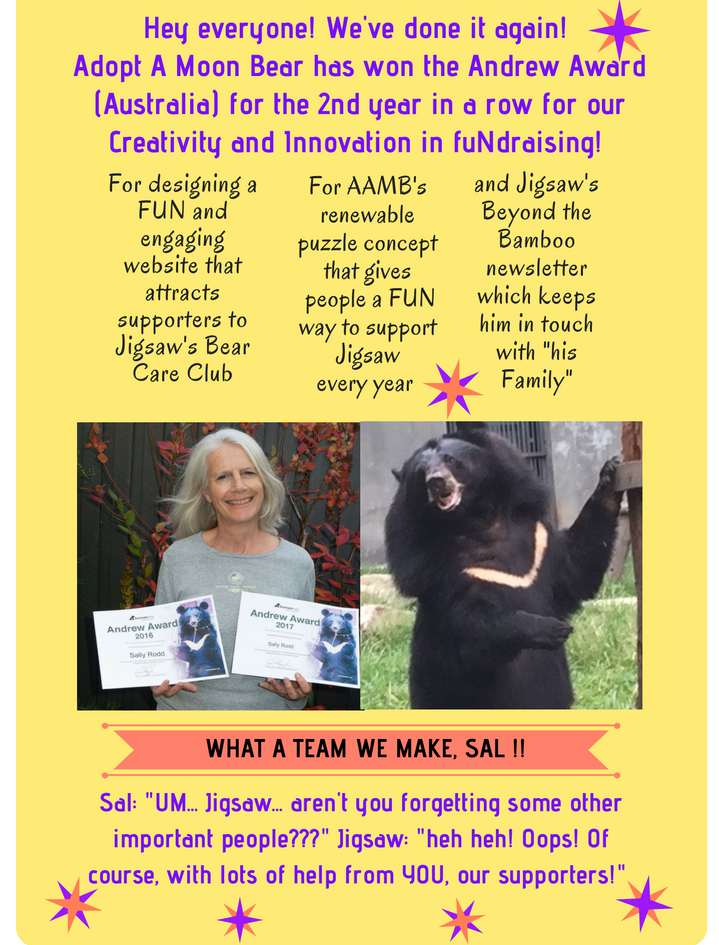table div table+table+table+table+table+table div table{width:100%;padding:0}table div table+table+table+table+table+table div table img{width:96.23%;padding:0;float:none}table div table+table+table+table+table+table div table td{width:100%;padding:0 1.88% 18px}/* styles */## Well you think our award would be big enough reason for a party ..... but wow! It's the 20th Anniversary for Animals Asia this year! Just think...I wouldn't be here writing Beyond the Bamboo news if it wasn't for the big heart and inspired vision of our founder Jill Robinson.

 table div table+table+table+table+table+table+table+table div table td,table.module-7{width:100%;padding:0}table div table+table+table+table+table+table+table+table div table{width:100%;float:none;margin-left:auto;margin-right:auto;padding:0}table div table+table+table+table+table+table+table+table div table a{border:0 none;text-decoration:none}table div table+table+table+table+table+table+table+table div table img{width:100%!important;border:0 none;text-decoration:none}/* styles */

## Would you like to meet one of those lucky first bears to be rescued. Here she is, still going strong in her mid 20's and loving life.

 table div table+table+table+table+table+table+table+table+table+table div table td,table.module-9{width:100%;padding:0}table div table+table+table+table+table+table+table+table+table+table div table{width:100%;float:none;margin-left:auto;margin-right:auto;padding:0}table div table+table+table+table+table+table+table+table+table+table div table a{border:0 none;text-decoration:none}table div table+table+table+table+table+table+table+table+table+table div table img{width:100%!important;border:0 none;text-decoration:none}/* styles */

## heh heh.... now back up there Jill mentioned the magic words "raising funds". Sal reminded me that for some of you it's that special (tax) time of year when you might be making some donations.... maybe I could help you spend it.. a very few examples below..table div table+table+table+table+table+table+table+table+table+table+table+table+table div table{width:100%;padding:0}table div table+table+table+table+table+table+table+table+table+table+table+table+table div table img{width:96.23%;padding:0;float:none}table div table+table+table+table+table+table+table+table+table+table+table+table+table div table td{width:100%;padding:0 1.88% 18px}/* styles */## And of course every donation goes towards making me look even more impressive in my personal puzzle!! Excitingly we galloped along after the last newsletter and are now at the HALF WAY mark with only 50 pieces to go! However I still need your help!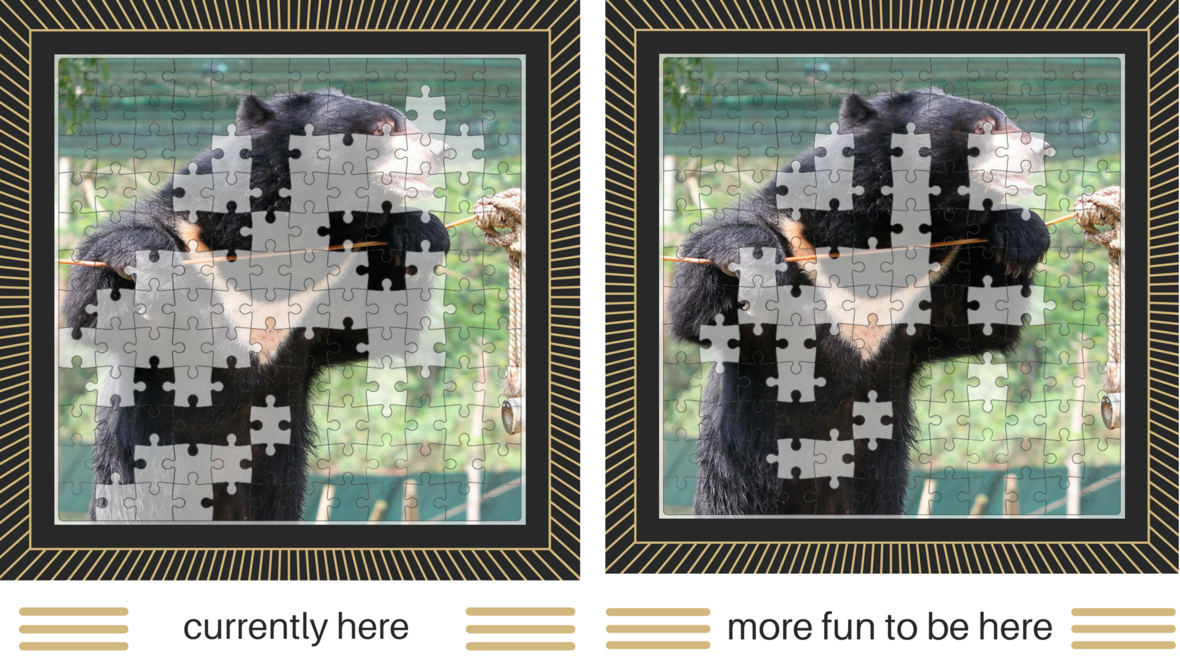FEEL FREE TO HELP ME ALONG THIS MONTH! DONATE NOW!

## Do you remember I am heading for this milestone too! It would be soooo cool to achieve our target in this special year, the 20th anniversary of Animals Asia. We are up around the \$95,000 mark so closing in!

 table.module-17{width:62.45%;padding:0}table div table+table+table+table+table+table+table+table+table+table+table+table+table+table+table+table+table+table div table{width:62.45%;float:none;margin-left:auto;margin-right:auto;padding:0}table div table+table+table+table+table+table+table+table+table+table+table+table+table+table+table+table+table+table div table a{border:0 none;text-decoration:none}table div table+table+table+table+table+table+table+table+table+table+table+table+table+table+table+table+table+table div table img{width:100%!important;border:0 none;text-decoration:none}table div table+table+table+table+table+table+table+table+table+table+table+table+table+table+table+table+table+table div table td{width:100%;padding:0}/* styles */
 table div table+table+table+table+table+table+table+table+table+table+table+table+table+table+table+table+table+table+table div table{width:100%;padding:0}table div table+table+table+table+table+table+table+table+table+table+table+table+table+table+table+table+table+table+table div table img{width:96.23%;padding:0;float:none}table div table+table+table+table+table+table+table+table+table+table+table+table+table+table+table+table+table+table+table div table td{width:100%;padding:0 1.88% 18px}/* styles */## While you're thinking about your donation... here's a Fun Bear Fact? Wouldn't you know... it's about .. FOOD! I wouldn't mind the job of preparing it each week but it might be a case of too much "chomp" and not enough "chop" if they let me in the kitchen.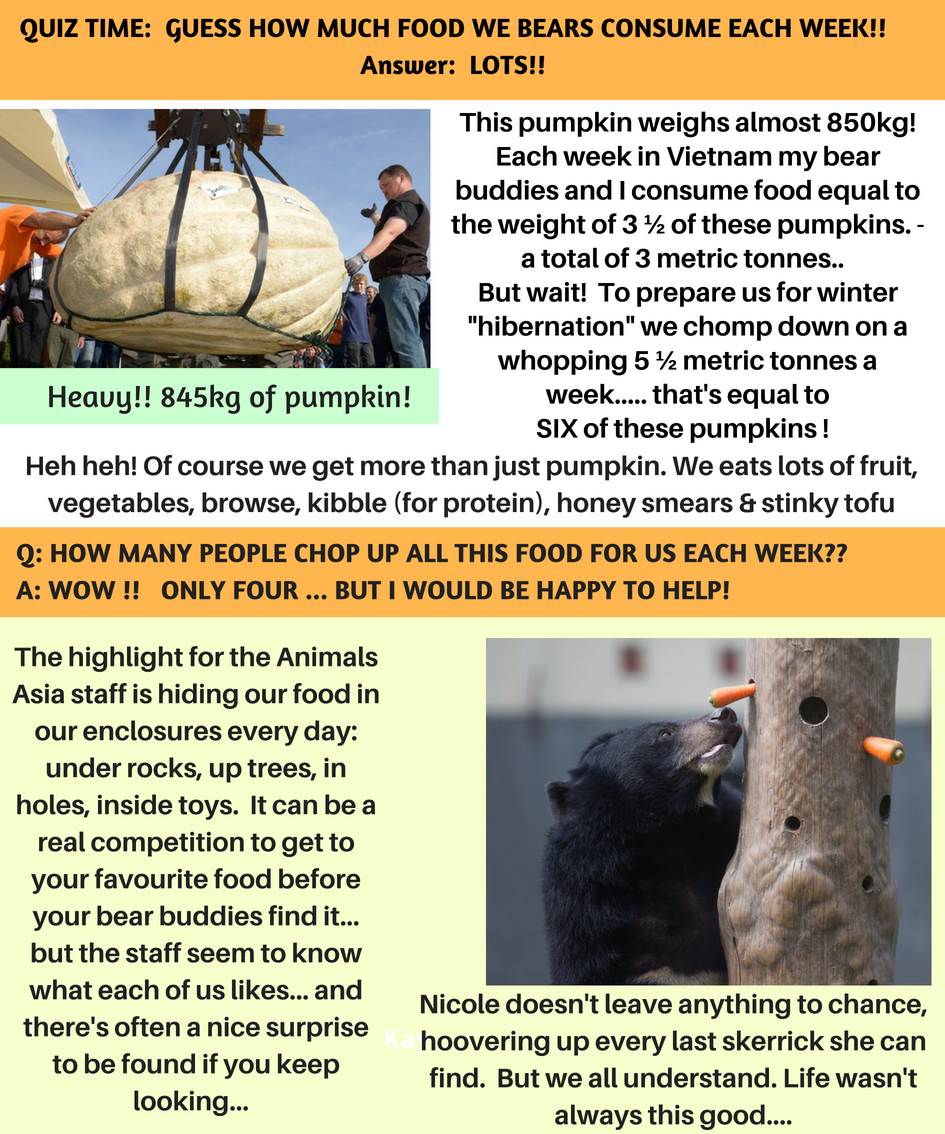CLICK HERE TO WATCH SOME FUN VIDEOS OF BEARS ENJOYING THE ENRICHMENT PROGRAMS!
 /* styles */

## Thanks everyone for reading my newsletter. I just want to finish with my little poem about why we bears are so grateful for the support you give us. Hope to hear from you soon... Love, YOUR bear, Jigsaw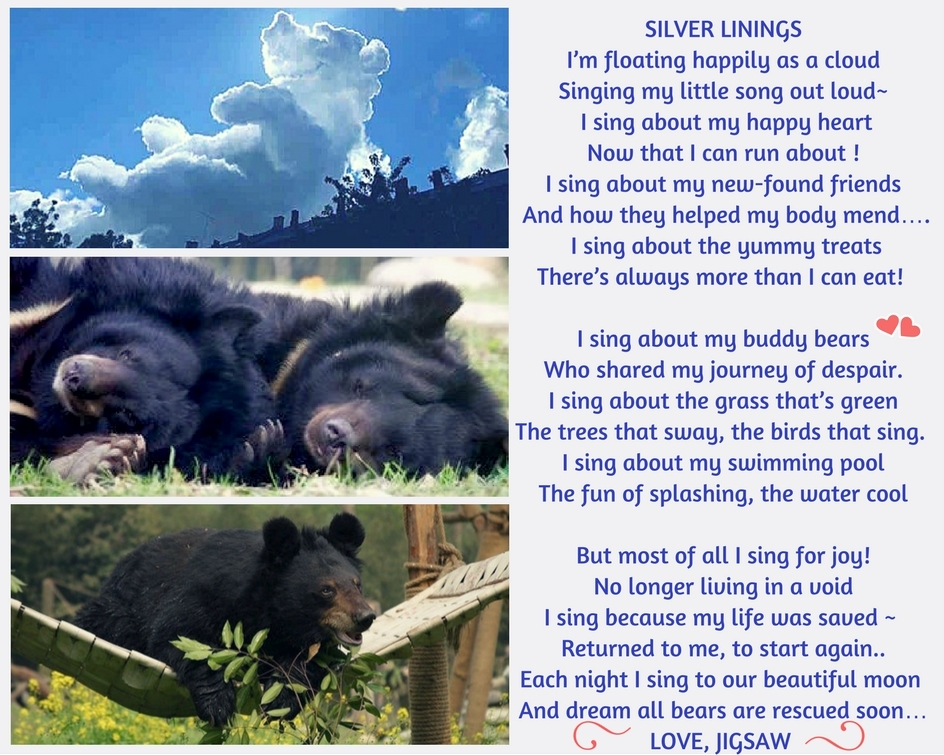table div table+table+table+table+table+table+table+table+table+table+table+table+table+table+table+table+table+table+table+table+table+table+table+table+table div table{width:100%;padding:0}table div table+table+table+table+table+table+table+table+table+table+table+table+table+table+table+table+table+table+table+table+table+table+table+table+table div table img{width:96.23%;padding:0;float:none}table div table+table+table+table+table+table+table+table+table+table+table+table+table+table+table+table+table+table+table+table+table+table+table+table+table div table td{width:100%;padding:0 1.88% 18px}/* styles */table.module-25{width:71.13%;padding:0}table div table+table+table+table+table+table+table+table+table+table+table+table+table+table+table+table+table+table+table+table+table+table+table+table+table+table div table{width:71.13%;float:none;margin-left:auto;margin-right:auto;padding:0}table div table+table+table+table+table+table+table+table+table+table+table+table+table+table+table+table+table+table+table+table+table+table+table+table+table+table div table a{border:0 none;text-decoration:none}table div table+table+table+table+table+table+table+table+table+table+table+table+table+table+table+table+table+table+table+table+table+table+table+table+table+table div table img{width:100%!important;border:0 none;text-decoration:none}table div table+table+table+table+table+table+table+table+table+table+table+table+table+table+table+table+table+table+table+table+table+table+table+table+table+table div table td{width:100%;padding:0}/* styles */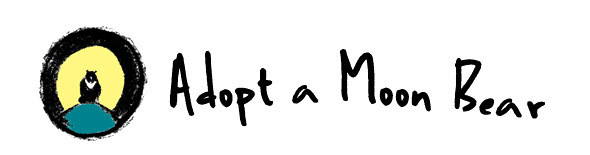table div table+table+table+table+table+table+table+table+table+table+table+table+table+table+table+table+table+table+table+table+table+table+table+table+table+table+table+table div table{width:100%;padding:0}table div table+table+table+table+table+table+table+table+table+table+table+table+table+table+table+table+table+table+table+table+table+table+table+table+table+table+table+table div table img{width:96.23%;padding:0;float:none}table div table+table+table+table+table+table+table+table+table+table+table+table+table+table+table+table+table+table+table+table+table+table+table+table+table+table+table+table div table td{width:100%;padding:0 1.88% 18px}/* styles */table.module-28{width:22.26%;padding:0}table div table+table+table+table+table+table+table+table+table+table+table+table+table+table+table+table+table+table+table+table+table+table+table+table+table+table+table+table+table div table{width:22.26%;float:none;margin-left:auto;margin-right:auto;padding:0}table div table+table+table+table+table+table+table+table+table+table+table+table+table+table+table+table+table+table+table+table+table+table+table+table+table+table+table+table+table div table a{border:0 none;text-decoration:none}table div table+table+table+table+table+table+table+table+table+table+table+table+table+table+table+table+table+table+table+table+table+table+table+table+table+table+table+table+table div table img{width:100%!important;border:0 none;text-decoration:none}table div table+table+table+table+table+table+table+table+table+table+table+table+table+table+table+table+table+table+table+table+table+table+table+table+table+table+table+table+table div table td{width:100%;padding:0}/* styles */
 Like   Tweet   Pin   +1